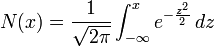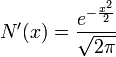Black-Scholes

[from Wikipedia]

The Black–Scholes model (pronounced /ˌblæk ˈʃoʊlz/) or Black–Scholes-Merton is a mathematical model of a financial market containing certain derivative investment instruments. From the model, one can deduce the Black–Scholes formula, which gives the price of European-style options. The formula led to a boom in options trading and the creation of theChicago Board Options Exchange. lt is widely used by options market participants. Many empirical tests have shown the Black–Scholes price is “fairly close” to the observed prices, although there are well-known discrepancies such as the “option smile”.

The model was first articulated by Fischer Black and Myron Scholes in their 1973 paper, “The Pricing of Options and Corporate Liabilities.” They derived a partial differential equation, now called the Black–Scholes equation, which governs the price of the option over time. The key idea behind the derivation was to perfectly hedge the option by buying and selling the underlying asset in just the right way and consequently “eliminate risk”. This hedge is called delta hedging and is the basis of more complicated hedging strategies such as those engaged in by Wall Street investment banks. The hedge implies there is only one right price for the option and it is given by the Black–Scholes formula.

Robert C. Merton was the first to publish a paper expanding the mathematical understanding of the options pricing model and coined the term Black–Scholes options pricing model. Merton and Scholes received the 1997 Nobel Prize in Economics (The Sveriges Riksbank Prize in Economic Sciences in Memory of Alfred Nobel) for their work. Though ineligible for the prize because of his death in 1995, Black was mentioned as a contributor by the Swedish academy.

Assumptions

The Black–Scholes model of the market for a particular stock makes the following explicit assumptions:

From these assumptions, Black and Scholes showed that “it is possible to create a hedged position, consisting of a long position in the stock and a short position in the option, whose value will not depend on the price of the stock.”

Several of these assumptions of the original model have been removed in subsequent extensions of the model. Modern versions account for changing interest rates (Merton, 1976), transaction costs and taxes (Ingersoll, 1976), and dividend payout.

Notation

Let

S, be the price of the stock (please note as below).
V(S,t), the price of a derivative as a function of time and stock price.
C(S,t) the price of a European call option and P(S,t) the price of a European put option.
K, the strike of the option.
r, the annualized risk-free interest ratecontinuously compounded.
μ, the drift rate of S, annualized.
σ, the volatility of the stock’s returns; this is the square root of the quadratic variation of the stock’s log price process.
t, a time in years; we generally use: now=0, expiry=T.
Π, the value of a portfolio.

Finally we will use N(x) which denotes the standard normal cumulative distribution function,.

N‘(x) which denotes the standard normal probability density function,.